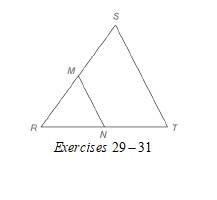Chapter 4.2, Problem 30EElementary Geometry For College St...

7th Edition
Alexander + 2 others
ISBN: 9781337614085

Solutions

Chapter
SectionElementary Geometry For College St...

7th Edition
Alexander + 2 others
ISBN: 9781337614085
Textbook Problem

In Exercises 29 to 31, M and N are the midpoints of sides R S ¯ and R T ¯ of ∆ R S T , respectively. Given: M N = x 2 + 5   S T = x ( 2 x + 5 ) Find: x , M N , S TTo determine

To find:

x, MN, and ST

For MN=x2+5

ST=x(2x+5)

Explanation

Calculation:

Given,

MN=x2+5

ST=x(2x+5)

M and N are the midpoints of sides RS¯ and RT¯ of RST, respectively.

A line segment that joins the midpoints of two sides of a triangle is parallel to the third side and has a length equal to one-half the length of the third side.

Thus,

MN=12ST

x2+5=12·x(2x+5)

Multiply; by 2 on both sides,

2(x2+5)=2·12·x(2x+5)

2(x2+5)=x(2x+5)

Apply the distributive property of multiplication over addition to the left and right of the above equation

Still sussing out bartleby?

Check out a sample textbook solution.

See a sample solution

The Solution to Your Study Problems

Bartleby provides explanations to thousands of textbook problems written by our experts, many with advanced degrees!

Get Started

Find more solutions based on key concepts